Courses

# Laplace Transform (Part - 1) - Mathematical Methods of Physics, UGC - NET Physics Physics Notes | EduRev

## Physics for IIT JAM, UGC - NET, CSIR NET

Created by: Akhilesh Thakur

## Physics : Laplace Transform (Part - 1) - Mathematical Methods of Physics, UGC - NET Physics Physics Notes | EduRev

The document Laplace Transform (Part - 1) - Mathematical Methods of Physics, UGC - NET Physics Physics Notes | EduRev is a part of the Physics Course Physics for IIT JAM, UGC - NET, CSIR NET.
All you need of Physics at this link: Physics

Laplace transform

The Laplace transform is a widely used integral transform with many applications in physics and engineering. Denoted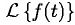, it is a linear operator of a function f(t) with a real argument t (t ≥ 0) that transforms it to a function F(s) with a complex argument s. This transformation is essentially bijective for the majority of practical uses; the respective pairs of f(t) and F(s) are matched in tables. The Laplace transform has the useful property that many relationships and operations over the originals f(t) correspond to simpler relationships and operations over the images F(s).  It is named after Pierre-Simon Laplace, who introduced the transform in his work on probability theory.

The Laplace transform is related to the Fourier transform, but whereas the Fourier transform expresses a function or signal as a series of modes of vibration (frequencies), the Laplace transform resolves a function into its moments.

Like the Fourier transform, the Laplace transform is used for solving differential and integral equations. In physics and engineering it is used for analysis of linear time-invariant systems such as electrical circuits, harmonic oscillators, optical devices, and mechanical systems. In such analyses, the Laplace transform is often interpreted as a transformation from the time-domain, in which inputs and outputs are functions of time, to the frequency-domain, where the same inputs and outputs are functions of complex angular frequency, in radians per unit time. Given a simple mathematical or functional description of an input or output to a system, the Laplace transform provides an alternative functional description that often simplifies the process of analyzing the behavior of the system, or in synthesizing a new system based on a set of specifications.

History

The Laplace transform is named after mathematician and astronomer Pierre-Simon Laplace, who used a similar transform (now called z transform) in his work on probability theory. The current widespread use of the transform came about soon after World War II although it had been used in the 19th century by Abel, Lerch, Heaviside and Bromwich. The older history of similar transforms is as follows. From 1744, Leonhard Euler investigated integrals of the form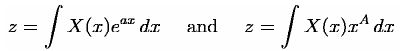as solutions of differential equations but did not pursue the matter very farJoseph Louis Lagrange was an admirer of Euler and, in his work on integrating probability density functions, investigated expressions of the form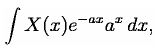which some modern historians have interpreted within modern Laplace transform theory.   These types of integrals seem first to have attracted Laplace's attention in 1782 where he was following in the spirit of Euler in using the integrals themselves as solutions of equations. However, in 1785, Laplace took the critical step forward when, rather than just looking for a solution in the form of an integral, he started to apply the transforms in the sense that was later to become popular. He used an integral of the form: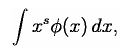akin to a Mellin transform, to transform the whole of a difference equation, in order to look for solutions of the transformed equation. He then went on to apply the Laplace transform in the same way and started to derive some of its properties, beginning to appreciate its potential power.

Laplace also recognised that Joseph Fourier's method of Fourier series for solving the diffusion equation could only apply to a limited region of space as the solutions were periodic. In 1809, Laplace applied his transform to find solutions that diffused indefinitely in space.

Formal definition

The Laplace transform of a function f(t), defined for all real numbers t ≥ 0, is the function F(s), defined by: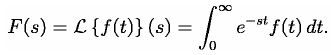The parameter s is a complex number:

s = σ + iω  with real numbers σ and ω.
The meaning of the integral depends on types of functions of interest. A necessary condition for existence of the integral is that f must be locally integrable on [0,∞). For locally integrable functions that decay at infinity or are of exponential type, the integral can be understood as a (proper) Lebesgue integral. However, for many applications it is necessary to regard it as a conditionally convergent improper integral at ∞. Still more generally, the integral can be understood in a weak sense, and this is dealt with below.
One can define the Laplace transform of a finite Borel measure μ by the Lebesgue integral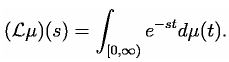An important special case is where μ is a probability measure or, even more specifically, the Dirac delta function. In operational calculus, the Laplace transform of a measure is often treated as though the measure came from a distribution function f. In that case, to avoid potential confusion, one often writes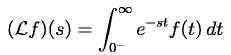where the lower limit of 0 is shorthand notation for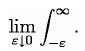This limit emphasizes that any point mass located at 0 is entirely captured by the Laplace transform. Although with the Lebesgue integral, it is not necessary to take such a limit, it does appear more naturally in connection with the Laplace–Stieltjes transform.

Probability theory

In pure and applied probability, the Laplace transform is defined as an expected value. If X is a random variable with probability density function f, then the Laplace transform of f is given by the expectation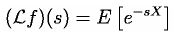By abuse of language, this is referred to as the Laplace transform of the random variable X itself. Replacing s by −t gives the moment generating function of X. The Laplace transform has applications throughout probability theory, including first passage times of stochastic processes such as Markov chains, and renewal theory.
Of particular use is the ability to recover the probability distribution function of a random variable X by means of the Laplace transform as follows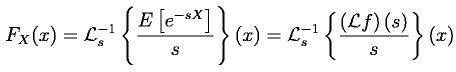Bilateral Laplace transform

When one says "the Laplace transform" without qualification, the unilateral or one-sided transform is normally intended. The Laplace transform can be alternatively defined as the bilateral Laplace transform or two-sided Laplace transform by extending the limits of integration to be the entire real axis. If that is done the common unilateral transform simply becomes a special case of the bilateral transform where the definition of the function being transformed is multiplied by the Heaviside step function.
The bilateral Laplace transform is defined as follows: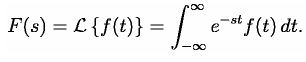Inverse Laplace transform

The inverse Laplace transform is given by the following complex integral, which is known by various names (the Bromwich integral, the Fourier-Mellin integral, and Mellin's inverse formula):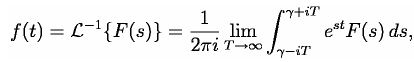where γ is a real number so that the contour path of integration is in the region of convergence of F(s). An alternative formula for the inverse Laplace transform is given by Post's inversion formula.

Region of convergence

If f is a locally integrable function (or more generally a Borel measure locally of bounded variation), then the Laplace transform F(s) of f converges provided that the limit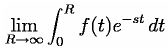exists. The Laplace transform converges absolutely if the integral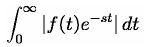exists (as a proper Lebesgue integral). The Laplace transform is usually understood as conditionally convergent, meaning that it converges in the former instead of the latter sense.
The set of values for which F(s) converges absolutely is either of the form Re(s) > a or else Re(s) ≥ a, where a is an extended real constant, −∞ ≤ a ≤ ∞. (This follows from the dominated convergence theorem.) The constant a is known as the abscissa of absolute convergence, and depends on the growth behavior of f(t). Analogously, the two-sided transform converges absolutely in a strip of the form a < Re(s) < b, and possibly including the lines Re(s) = a or Re(s) = b. The subset of values of s for which the Laplace transform converges absolutely is called the region of absolute convergence or the domain of absolute convergence. In the two-sided case, it is sometimes called the strip of absolute convergence. The Laplace transform is analytic in the region of absolute convergence.
Similarly, the set of values for which F(s) converges (conditionally or absolutely) is known as the region of conditional convergence, or simply the region of convergence (ROC). If the Laplace transform converges (conditionally) at s = s0, then it automatically converges for all s with Re(s) > Re(s0). Therefore the region of convergence is a half-plane of the form Re(s) > a, possibly including some points of the boundary line Re(s) = a. In the region of convergence Re(s) > Re(s0), the Laplace transform of f can be expressed by integrating by parts as the integral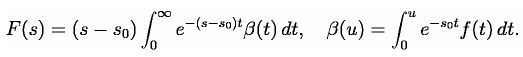That is, in the region of convergence F(s) can effectively be expressed as the absolutely convergent Laplace transform of some other function. In particular, it is analytic.
A variety of theorems, in the form of Paley–Wiener theorems, exist concerning the relationship between the decay properties of f and the properties of the Laplace transform within the region of convergence.
In engineering applications, a function corresponding to a linear time-invariant (LTI) system is stable if every bounded input produces a bounded output. This is equivalent to the absolute convergence of the Laplace transform of the impulse response function in the region Re(s) ≥ 0. As a result, LTI systems are stable provided the poles of the Laplace transform of the impulse response function have negative real part.

Properties and theorems

The Laplace transform has a number of properties that make it useful for analyzing linear dynamical systems. The most significant advantage is that differentiation and integration become multiplication and division, respectively, by s (similarly to logarithms changing multiplication of numbers to addition of their logarithms). Because of this property, the Laplace variable s is also known as operator variable in the L domain: either derivative operator or (for s−1) integration operator. The transform turns integral equations and differential equations to polynomial equations, which are much easier to solve. Once solved, use of the inverse Laplace transform reverts to the time domain.
Given the functions f(t) and g(t), and their respective Laplace transforms F(s) and G(s):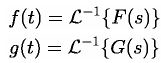the following table is a list of properties of unilateral Laplace transform:

Properties of the unilateral Laplace transform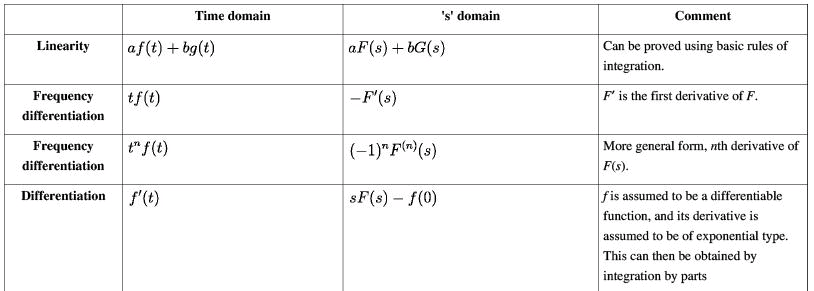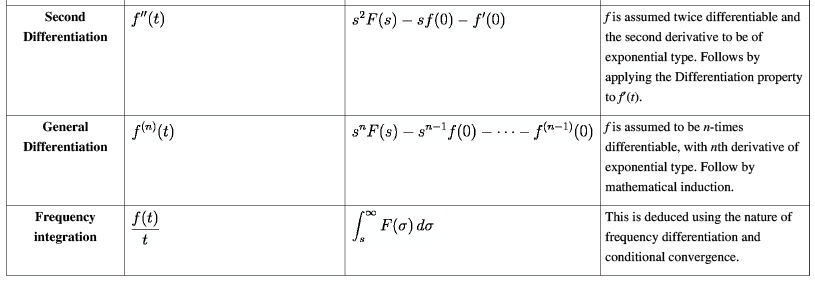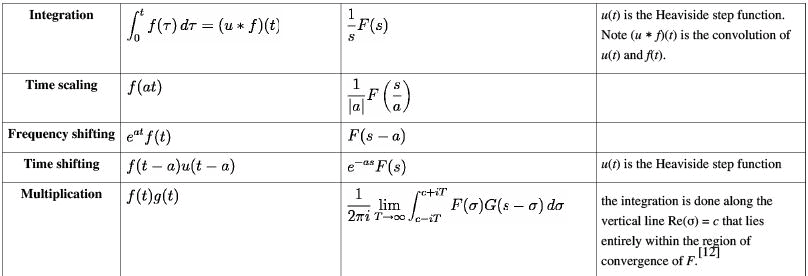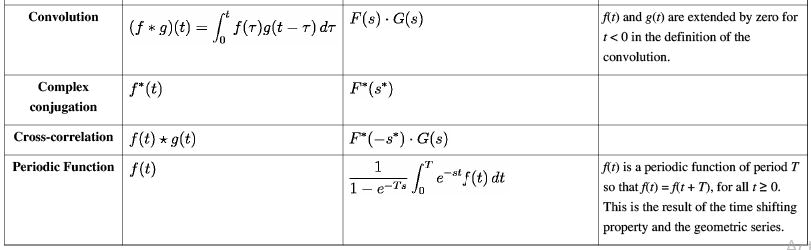•  Initial value theorem: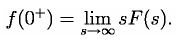• Final value theorem: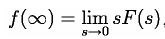if all poles of sF(s) are in the left half-plane.
The final value theorem is useful because it gives the long-term behaviour without having to perform partial fraction decompositions or other difficult algebra. If a function has poles in the right-hand plane or on the imaginary axis, (e.g. eor sin(t), respectively) the behaviour of this formula is undefined.

Relation to moments

The quantities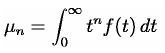are the moments of the function f. Note by repeated differentiation under the integral,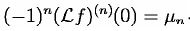This is of special significance in probability theory, where the moments of a random variable X are given by the expectation values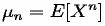Then the relation holds: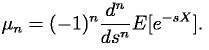Proof of the Laplace transform of a function's derivative

It is often convenient to use the differentiation property of the Laplace transform to find the transform of a function's derivative. This can be derived from the basic expression for a Laplace transform as follows: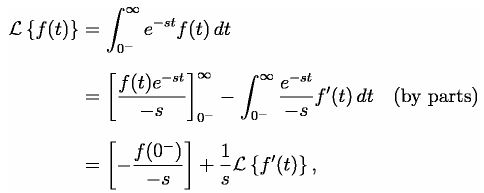yielding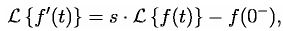and in the bilateral case,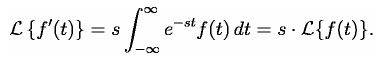The general result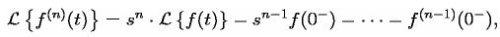where fn is the nth derivative of f, can then be established with an inductive argument.

Evaluating improper integrals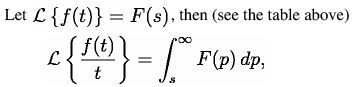or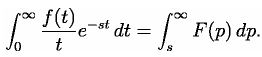Letting s → 0, we get the identity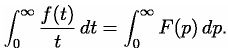For example,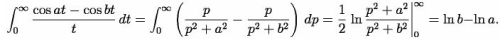Another example is Dirichlet integral.

Relationship to other transforms

Laplace–Stieltjes transform

The (unilateral) Laplace–Stieltjes transform of a function g : R → R is defined by the Lebesgue–Stieltjes integral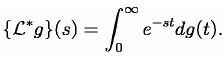The function g is assumed to be of bounded variation. If g is the antiderivative of f: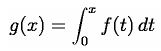then the Laplace–Stieltjes transform of g and the Laplace transform of f coincide. In general, the Laplace–Stieltjes transform is the Laplace transform of the Stieltjes measure associated to g. So in practice, the only distinction between the two transforms is that the Laplace transform is thought of as operating on the density function of the measure, whereas the Laplace–Stieltjes transform is thought of as operating on its cumulative distribution function.

Fourier transform

The continuous Fourier transform is equivalent to evaluating the bilateral Laplace transform with imaginary argument s = iω or s = 2πfi :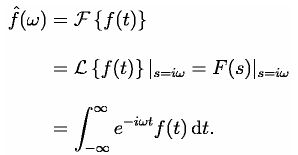This definition of the Fourier transform requires a prefactor of 1/2π on the reverse Fourier transform. This relationship between the Laplace and Fourier transforms is often used to determine the frequency spectrum of a signal or dynamical system.
The above relation is valid as stated if and only if the region of convergence (ROC) of F(s) contains the imaginary axis, σ = 0. For example, the function f(t) = cos(ω 0t) has a Laplace transform F(s) = s/(s+ ω02) whose ROC is Re(s) > 0. As s = iω is a pole of F(s), substituting s = iω in F(s) does not yield the Fourier transform of f(t)u(t), which is proportional to the Dirac delta-function δ(ω-ω 0).
However, a relation of the form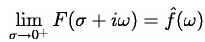holds under much weaker conditions. For instance, this holds for the above example provided that the limit is understood as a weak limit of measures (see vague topology). General conditions relating the limit of the Laplace transform of a function on the boundary to the Fourier transform take the form of Paley-Wiener theorems.

Mellin transform

The Mellin transform and its inverse are related to the two-sided Laplace transform by a simple change of variables.
If in the Mellin transform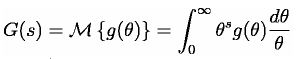we set θ = e−t we get a two-sided Laplace transform.

Z-transform

The unilateral or one-sided Z-transform is simply the Laplace transform of an ideally sampled signal with the substitution of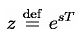where T = 1/f s is the sampling period (in units of time e.g., seconds) and f s is the sampling rate (in samples per second or hertz)

Let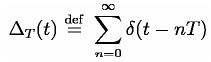be a sampling impulse train (also called a Dirac comb) and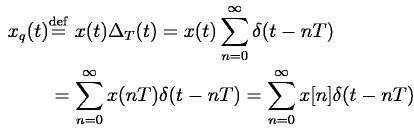be the continuous-time representation of the sampled x(t)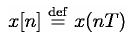The Laplace transform of the sampled signal xqt  is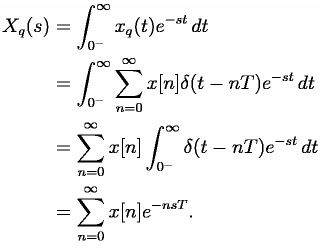This is precisely the definition of the unilateral Z-transform of the discrete function x[n]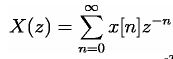with the substitution of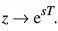Comparing the last two equations, we find the relationship between the unilateral Z-transform and the Laplace transform of the sampled signal: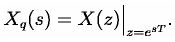The similarity between the Z and Laplace transforms is expanded upon in the theory of time scale calculus.

Borel transform

The integral form of the Borel transform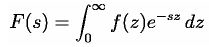is a special case of the Laplace transform for f an entire function of exponential type, meaning that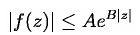for some constants A and B. The generalized Borel transform allows a different weighting function to be used, rather than the exponential function, to transform functions not of exponential type. Nachbin's theorem gives necessary and sufficient conditions for the Borel transform to be well defined.

159 docs

,

,

,

,

,

,

,

,

,

,

,

,

,

,

,

,

,

,

,

,

,

,

,

,

;